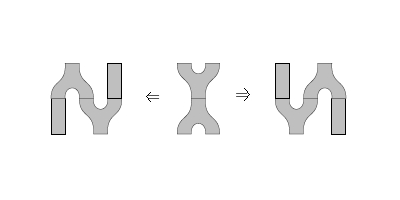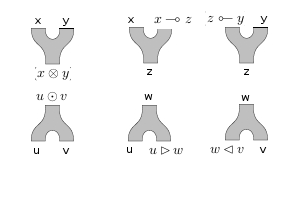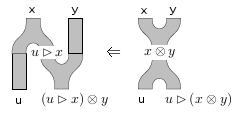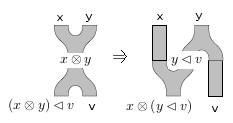## November 17, 2017

### Star-autonomous Categories are Pseudo Frobenius Algebras

#### Posted by Mike ShulmanA little while ago I talked about how multivariable adjunctions naturally form a polycategory: a structure like a multicategory, but in which codomains as well as domains can involve multiple objects. Now I want to talk about some structures we can define inside this polycategory $MVar$.

What can you define inside a polycategory? Well, to start with, a polycategory has an underlying multicategory, consisting of the arrows with unary target; so anything we can define in a multicategory we can define in a polycategory. And the most basic thing we can define in a multicategory is a monoid object — in fact, there are some senses in which this is the canonical thing we can define in a multicategory.

So what is a monoid object in $MVar$?

Well, actually it’s more interesting to ask about pseudomonoid objects, using the 2-categorical structure of $MVar$. In this case what we have is an object $A$, a (0,1)-variable adjunction $i:()\to A$ (which, recall, is just an object $i\in A$), and a (2,1)-variable adjunction $m:(A,A)\to A$, together with coherent associativity and unit isomorphisms. The left adjoint part of $m$ is a functor $A\times A\to A$, and the associativity and unit isomorphisms then make $A$ into a monoidal category. And to say that this functor extends to a multivariable adjunction is precisely to say that $A$ is a closed monoidal category, i.e. that its tensor product has a right adjoint in each variable:

$A(x\otimes y,z) \cong A(y, x\multimap z) \cong A(x, z \;⟜\; y)$

Similarly, we can define braided pseudomonoids and symmetric pseudomonoids in any 2-multicategory, and in $MVar$ these specialize to braided and symmetric closed monoidal categories.

Now, what can we define in a polycategory that we can’t define in a multicategory? The most obvious monoid-like thing that involves multiple objects in a codomain is a comonoid. So what is a pseudo-comonoid in $MVar$?

I think this question is easiest to answer if we use the duality of $MVar$ to turn everything around. So a pseudo-comonoid structure on a category $A$ is the same as a pseudo-monoid structure on $A^{op}$. In terms of $A$, that means it’s a monoidal structure that’s co-closed, i.e. the tensor product functor has a left adjoint in each variable:

$A(x, y \odot z) \cong A(y \rhd x, z) \cong A(x \lhd z , y).$

The obvious next thing to do is to mix a monoid structure with a comonoid structure. In general, there’s more than one way to do that: we could think about bimonoids, Hopf monoids, or Frobenius monoids. However, while all of these can be defined in any symmetric monoidal category (or PROP), in a polycategory, bimonoids and Hopf monoids don’t make sense, because their axioms involve composing along multiple objects at once, whereas in a polycategory we are only allowed to compose along one object at a time.

Frobenius algebras, however, make perfect sense in a polycategory. If you look at the usual definition in a monoidal category, you can see that the axioms only involve composing along one object at once; when they’re written topologically that corresponds to the “absence of holes”.

So what is a pseudo Frobenius algebra in $MVar$? Actually, let’s ask a more general question first: what is a lax Frobenius algebra in $MVar$? By a lax Frobenius algebra I mean an object with a pseudo-monoid structure and a pseudo-comonoid structure, together with not-necessarily invertible “Frobenius-ator” 2-cellssatisfying some coherence axioms, which can be found for instance in this paper (pages 52-55). This isn’t quite as scary as it looks; there are 20 coherence diagrams listed there, but the first 2 are the associativity pentagons for the pseudomonoid and pseudo-comonoid, while the last 8 are the unit axioms for the pseudomonoid and pseudo-comonoid (of which the $17^{\mathrm{th}}$ and $18^{\mathrm{th}}$ imply the other 6, by an old observation of Kelly). Of the remaining 10 axioms, 6 assert compatibility of the Frobenius-ators with the associativities, while 4 assert their compatibility with the units.

Now, to work out what a lax Frobenius algebra in $MVar$ is, we need to figure out what (2,2)-variable adjunctions $(A,A)\to (A,A)$ those pictures represent. To work out what these functors are, I find it helpful to draw the monoid and comonoid structures with all the possible choices for input/output:By the mates correspondence, to characterize a 2-cell in $MVar$ it suffices to consider one of the functors involved in the multivariable adjunctions, which means we should pick one of the copies of $A$ to be the “output” and consider all the others as the “input”. I find it easier to pick different copies of $A$ for the two Frobenius-ators. For the first one, let’s pick the second copy of $A$ in the codomain; this givesIn the domain of the 2-cell, on the right, $x$ and $y$ come in and get combined into $x\otimes y$, and then that gets treated as $w$ and gets combined with $u$ coming in from the lower-left to give $u\rhd (x\otimes y)$. In the codomain of the 2-cell, on the left, first $x$ gets combined with $u$ to give $u\rhd x$, then that gets multiplied with $y$ to give $(u\rhd x) \otimes y$. Thus, the first Frobenius-ator is

$u\rhd (x\otimes y)\to (u\rhd x) \otimes y.$

For the second Frobenius-ator, let’s dually pick the first copy of $A$ in the codomain to be the output:Thus the second Frobenius-ator is

$(x\otimes y)\lhd v \to x\otimes (y\lhd v).$

What is this? Well, let’s take mates once with respect to the co-closed monoidal structure to reexpress both Frobenius-ators in terms of $\otimes$ and $\odot$. The first gives

$(u \odot x) \otimes y \to u \odot (u\rhd ((u \odot x) \otimes y)) \to u\odot ((u\rhd (u\odot x)) \otimes y) \to u \odot (x\otimes y).$

and the second dually gives

$x \otimes (y\odot v) \to ((x \otimes (y\odot v)) \lhd v) \odot v \to (x \otimes ((y\odot v) \lhd v)) \odot v \to (x\otimes y)\odot v.$

These two transformations $(u \odot x) \otimes y \to u \odot (x\otimes y)$ and $x \otimes (y\odot v) \to (x\otimes y)\odot v$ have exactly the shape of the “linear distributivity” transformations in a linearly distributive category! (Remember from last time that linearly distributive categories are the “representable” version of polycategories.) The latter are supposed to satisfy their own coherence axioms, which aren’t listed on the nLab, but if you look up the original Cockett-Seely paper and count them there are… 10 axioms… 6 asserting compatibility with associativity of $\otimes$ and $\odot$, and 4 asserting compatibility with the unit. In other words,

A lax Frobenius algebra in $MVar$ is precisely a linearly distributive category! (In which $\otimes$ is closed and $\odot$ is co-closed.)

Note that this is at least an approximate instance of the microcosm principle. (I have to admit that I have not actually checked that the two groups of coherence axioms coincide under the mates correspondence, but I find it inconceivable that they don’t.)

The next thing to ask is what a pseudo Frobenius algebra is, i.e. what it means for the Frobenius-ators to be invertible. If you’ve come this far (or if you read the title of the post) you can probably guess the answer: a $\ast$-autonomous category, i.e. a linearly distributive category in which all objects have duals (in the polycategorical sense I defined in the first post).

First note that in a $\ast$-autonomous category, $\otimes$ is always closed and $\odot$ is co-closed, with $(x\multimap z) = (x^\ast \odot z)$ and $(u\rhd w) = (u^\ast \otimes w)$ and so on. With these definitions, the Frobenius-ators become just associativity isomorphisms:

$u\rhd (x\otimes y) = u^\ast \otimes (x\otimes y) \cong (u^\ast \otimes x) \otimes y = (u\rhd x) \otimes y.$

$(x\otimes y)\lhd v = (x\otimes y)\otimes v^\ast \cong x\otimes (y\otimes v^\ast) = x\otimes (y\lhd v).$

Thus, an $\ast$-autonomous category is a pseudo Frobenius algebra in $MVar$. Conversely, if $A$ is a pseudo Frobenius algebra in $MVar$, then letting $x=i$ be the unit object of $\otimes$, we have

$u\rhd y \cong u\rhd (i\otimes y) \cong (u\rhd i) \otimes y$

giving an isomorphism

$A(y, u\odot v) \cong A(u\rhd y, v) \cong A((u\rhd i) \otimes y, v).$

Thus $u\rhd i$ behaves like a dual of $u$, and with a little more work we can show that it actually is. (I’m totally glossing over the symmetric/non-symmetric distinction here; in the non-symmetric case one has to distinguish between left and right duals, blah blah blah, but it all works.) So

A pseudo Frobenius algebra in $MVar$ is precisely a $\ast$-autonomous category!

The fact that there’s a relationship between Frobenius algebras and $\ast$-autonomous categories is not new. In this paper, Brian Day and Ross Street showed that pseudo Frobenius algebras in $Prof$ can be identified with “pro-$\ast$-autonomous categories”, i.e. promonoidal categories that are $\ast$-autonomous in a suitable sense. In this paper Jeff Egger showed that Frobenius algebras in the $\ast$-autonomous category Sup of suplattices can be identified with $\ast$-autonomous cocomplete posets. And Jeff has told me personally that he also noticed that lax Frobenius algebras correspond to mere linear distributivity. (By the way, the above characterization of $\ast$-autonomous categories as closed and co-closed linearly distributive ones such that certain transformations are invertible is due to Cockett and Seely.)

What’s new here is that the pseudo Frobenius algebras in $MVar$ are exactly $\ast$-autonomous categories — not pro, not posets, not cocomplete.

There’s more that could be said. For instance, it’s known that Frobenius algebras can be defined in many different ways. One example is that instead of giving an algebra and coalgebra structure related by a Frobenius axiom, we could give just the algebra structure along with a compatible nondegenerate pairing $A\otimes A \to I$. This is also true for pseudo Frobenius algebras in a polycategory, and in $MVar$ such a pairing $(A,A) \to ()$ corresponds to a contravariant self-equivalence $(-)^\ast : A\simeq A^{op}$, leading to the perhaps-more-common definition of star-autonomous category involving such a self-duality. And so on; but maybe I’ll stop here.

Posted at November 17, 2017 7:28 PM UTC

TrackBack URL for this Entry:   https://golem.ph.utexas.edu/cgi-bin/MT-3.0/dxy-tb.fcgi/2996

### Re: Star-autonomous categories are pseudo Frobenius algebras

What I find fascinating about this is that, to the logician, polycategories are very appealing as they behave very much like sequents. But that’s not what is happening here: the polyarrows represent inference rules (several rules per polyarrow).

I can’t say I have quite wrapped my head around it yet. But it’s certainly appealing.

Posted by: Arnaud Spiwack on November 18, 2017 11:09 AM | Permalink | Reply to this

### Re: Star-autonomous categories are pseudo Frobenius algebras

That’s the magic of the microcosm principle! The operations on a structure (e.g. the multiplication in a monoid $A$) become the morphisms in a categorified version of the structure (e.g. a morphism $A\otimes A\to A$ in a monoidal category). Here, the operations on a $\ast$-autonomous category (inference rules) become morphisms in a 2-polycategory.

From a syntactic point of view, it’s like a logical framework: the judgments of the object-language become types of the logical framework, and the inference rules of the object-language become terms of the logical framework.

Posted by: Mike Shulman on November 18, 2017 11:08 PM | Permalink | Reply to this

### Re: Star-autonomous categories are pseudo Frobenius algebras

It makes sense, but I can’t quite convince myself that this is what is happening here. I think it boils down to: is this the “right” category to speak about categorical logic, or did we get to star-autonomous categories by coincidence? (the fact that *-autonomous categories are so expressive is a very good sign though) How can I, for instance, define a cartesian-closed category as a structure in the 2-polycategory of multivariate adjunctions (we’ve seen symmetric monoidal closed categories, so far, and my intuition fails me for cartesian-closed ones, surely we have to use something related to the cartesian product of categories, as with ordinary adjunctions, but is there a natural way to do it in this framework?)

More directly on the microcosm principle: can we define a polycategory as a structure in the 2-polycategory of multivariate adjunctions?

Posted by: Arnaud Spiwack on November 19, 2017 8:29 AM | Permalink | Reply to this

### Re: Star-autonomous categories are pseudo Frobenius algebras

I’ve never claimed that any category is the right one to speak about categorical logic! The microcosm principle generally matches up one microcosm with one macrocosm; there’s no reason to expect a single macrocosm to suffice for all possible microcosms. In particular, I wouldn’t expect there to be any way to define a cartesian closed category in $MVar$; the cartesian product of categories isn’t really visible in $MVar$.

It is, I admit, a little unsatisfying that $\ast$-autonomous categories are “representable” but the structure that we define them in is not. True, there are some $\ast$-autonomous 2-categories closely related to $MVar$, such as $Sup$ (which it contains) and $Chu(Cat,Set)$ (which contains it), and Frobenius pseudomonoids in these are either specializations or generalizations of $\ast$-autonomous categories.

However, I would also argue it’s actually in line with other instances of the microcosm principle that the microcosm object is “strong” ($\ast$-autonomous category, i.e. representable polycategory with duals) but the macrocosm is “weak” (2-polycategory, not $\ast$-autonomous 2-category). Usually this “weakness” manifests itself as an up to isomorphism version of the microcosm, but there are also other instances where it manifests as an (even weaker) lax or multicategorical version, such as duoidal categories and intercategories.

Posted by: Mike Shulman on November 19, 2017 10:55 AM | Permalink | Reply to this

### Re: Star-autonomous Categories are Pseudo Frobenius Algebras

I think there is a small typo in

“Well, let take mates once with respect to the co-closed monoidal structure to reexpress both Frobenius-ators in terms of $\otimes$ and $\odot$. The first gives $(u \odot x) \otimes y \to u \odot (u\rhd ((u \odot x) \otimes y)) \to u\odot ((u\rhd (x\odot x)) \otimes y) \to u \odot (x\otimes y).$

Where the $(x \odot x)$ seems to be $(u \odot x)$.

Posted by: Mario Román on November 4, 2020 9:25 AM | Permalink | Reply to this

### Re: Star-autonomous Categories are Pseudo Frobenius Algebras

Posted by: Mike Shulman on November 7, 2020 1:43 AM | Permalink | Reply to this

Post a New Comment